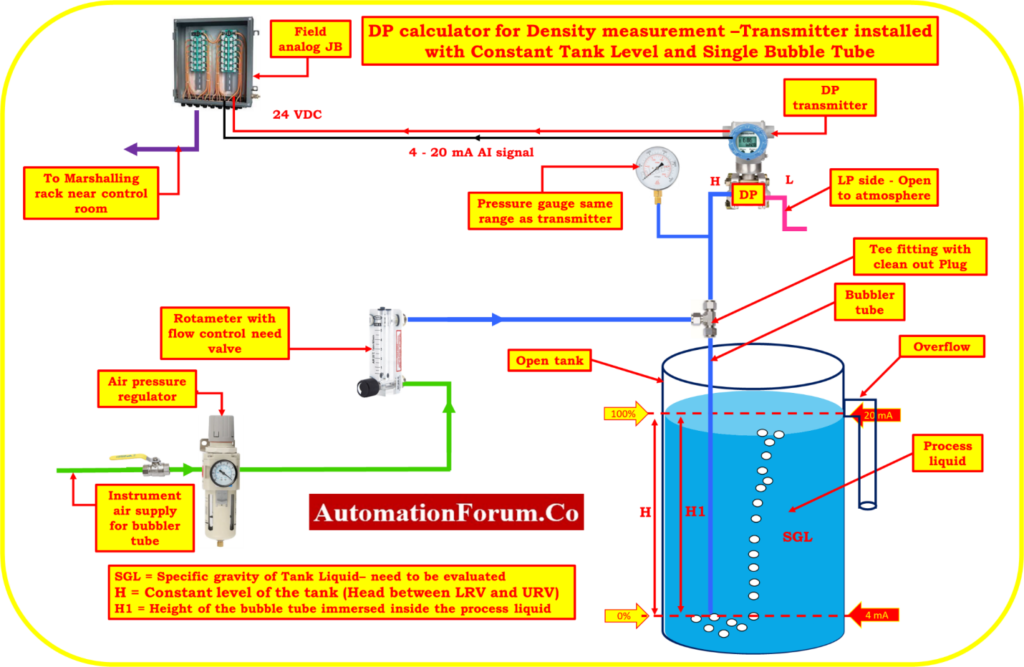# DP calculator for Density measurement – transmitter installed With Constant Tank Level and single Bubble Tube## How to Measure Density using DP Transmitter?

A DP (Differential Pressure) calculator is used to measure the density of fluids by calculating the difference in pressure between two points in the fluid. When a constant tank level and a single bubble tube are used with a DP calculator, it can accurately measure the density of the fluid. The transmitter installed in this system sends the measurement data to a monitoring system.

The constant tank level ensures that the fluid level in the tank remains consistent, allowing for more accurate and precise measurements. The single bubble tube provides a simple and effective way to measure the density of the fluid. The DP calculator is commonly used in various industries for fluid density measurements, including chemical, oil and gas, and food processing. Overall, the DP calculator with constant tank level and single bubble tube is a valuable tool for measuring the density of fluids and ensuring the efficiency and accuracy of industrial processes.

H1 = Height of the bubble tube immersed inside the process liquid

SGL = Specific gravity of the liquid – need to be evaluated

SG1 = Minimum Specific gravity of the liquid

SG2 = Maximum Specific gravity of the liquid

H = Constant level of the tank (Head between LRV and URV)

Calibrated Range of the transmitter = LRV to URV

Where:

HW = Equivalent Head level pressure of Water

## Formula for Span value calculation

Span value of the transmitter = (H)*(SG2 – SG1)

## Formula for LRV estimation

In order to determine the DP, it is necessary to assume that the tank was filled to the minimum specific gravity required for the LRV calculation.

Equivalent Head level pressure of Water (HW) at Lower range value

HW at LRV = (H*SG1)  = Suppression

## Formula for URV estimation

In order to determine the DP, it is necessary to assume that the tank was filled to the maximum specific gravity required for the URV calculation.

Equivalent Head level pressure of Water (HW) at Higher range value

HW at URV = (H*SG2)

## DP calculator

This below calculator used to calculate the Span value, LRV and URV of the transmitter for calibration in process liquid density measurement with constant tank level.